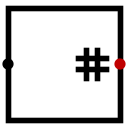#Bit-Addierer

 Bibliothek: Arithmetic Eingeführt: 2.6.0 Aussehen:## Verhalten

The component determines how many 1 bits are in its input(s) and emits the total number of 1 bits on its output. For example, given the 8-bit input 10011101, the output would be 5, since there are five 1-bits in the input (the first, the last, and a string of three bits in the middle).

If any of the input bits are floating or error values, then the output will contain error bits in the output corresponding to the range of possible outputs depending on whether those floating/error values are counted as zeroes or ones. For instance, if the 14-bit input is 111x10110x1101, then the output must be at least 9 (if the x's are interpreted as zeroes) and at most 11 (if they are interpreted as ones). Thus, the output will be 10EE: The upper two bits will be 1 and 0 since all integers between 9 and 11 have 1 and 0 as their top two bits, but the lower two bits are EE since integers between 9 and 11 vary within these bits.

## Pins

West edge (inputs, bit width matches Data Bits attribute)
The inputs whose 1 bits are to be counted. The number of inputs is based on the Number of Inputs attribute.
East edge (output, bit width computed as described below)
The number of input bits which are 1. The bit width of the output is the minimum number of bits to store the maximum possible value (which would be the product of the Data Bits attribute and the Number of Inputs attribute).

## Attribute

When the component is selected or being added, the digits '0' through '9' alter its Number of Inputs attribute and Alt-0 through Alt-9 alter its Data Bits attribute.

Data Bits
The bit width of the input(s).
Number of Inputs
The number of input values.

Keines.

## Verhalten des Textwerkzeugs

Keines.

Zurück zur Bibliotheksreferenz